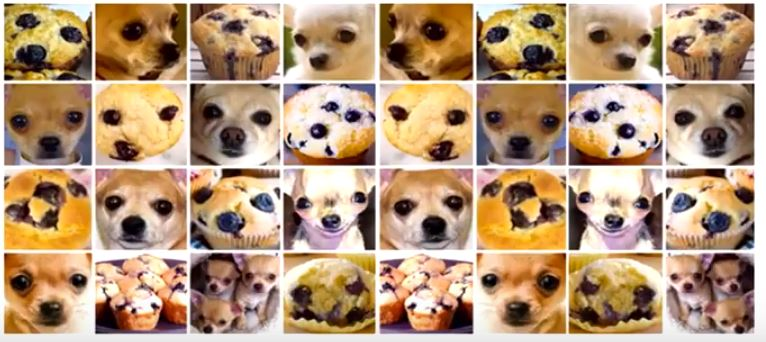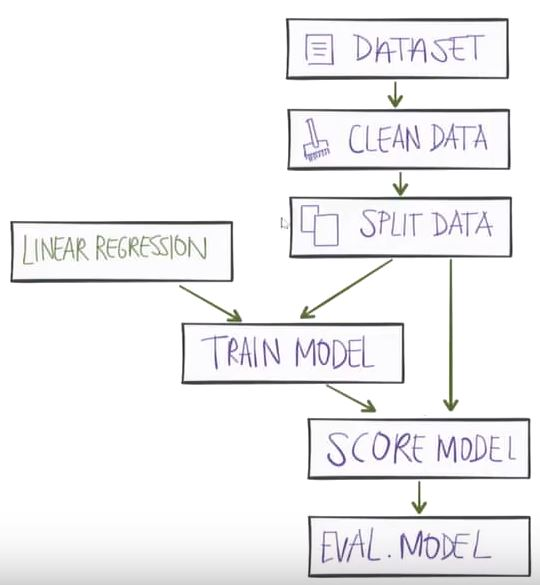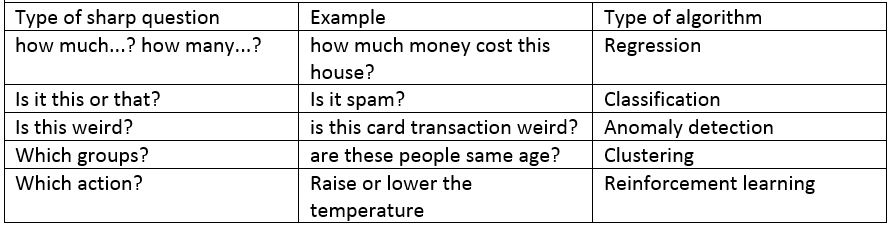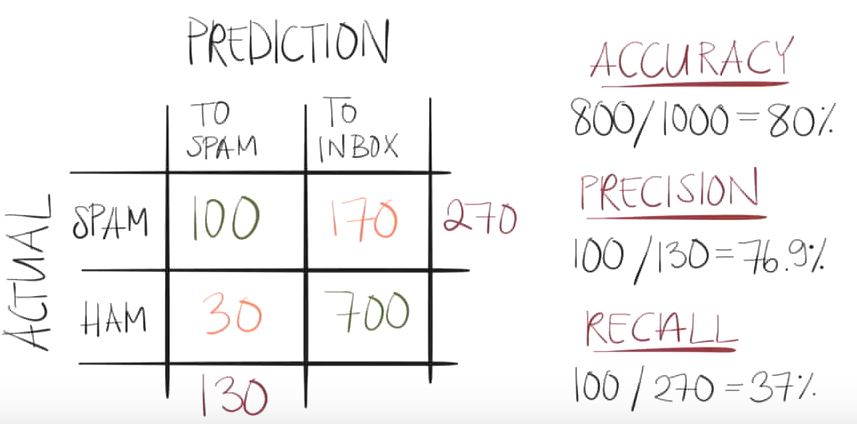# Understanding machine learning

I have watched this video from @TessFerrandez: Machine Learning for Developers.

The video explains how the process of building a machine learning solution is. She explains it in plain English and with very nice examples easy to remind the concepts.

The video helped me to link a lot of technical ideas explained in the courses with a natural flow. Now all make sense to me.

# When do I need a machine learning solution?

Imagine that you have this catalogue of pictures:and that you want to identify when a picture has a muffin or a chihuahua.

The traditional way to do it is using “if / else” sentences, like for example:The results are not going to be good, why?

• The problem is more complex than the basic questions you are doing and it requires thounsand of combinations of conditional sentences.
• To find the right sequence of conditional sentences can take years.

Here, is when machine learning techniques can help you. At the end of the day, it’s a different approach to find a solution to a complex problem.

# What are the steps to perform a machine learning solution?

The basic steps to build a machine learning solution are:## 1.- Ask a sharp question:At the end of the day, depending on the question you ask, you will use a different machine learning techniques.

What type of machine learning technique can I use? Well there are so many of them, but these are the basic ones:

• Supervised learning : learning model based on a set of labeled examples. For instance you want to identify when there is a cat in a picture and you use a set of pictures where you know that there are indeed cats.
• Unsupervised learning: think about the a data set of population where you use a clustering algorithm to classify the people in five different groups, but we do not say in which type of groups. For instance when we are looking for movies recommendations and suddenly there is a pattern identified by age (which initially we did not know it was a cluster of relevant data that we could cluster or classify)
• Reinforcement : it uses the feedback to make decisions. For instance, a system that measures the temperature, then it compares with the target temperature and finally raise or lower the temperature. This reminds me to the servo-systems and fuzzy logic used at electronic level when I studied electronic engineering.

## 2.- Collect data

look for databanks, there are so many on the internet. For sure if you want precise trading data from a good bunch of markets and thousands of parameters, you will have to pay for it.

## 3.- Explore data: relevant, important and simple.

• Relevant: determine features, define relevant features, discard irrelevant features.
• Important: define important data.
• Simple: it has to be simple (for instance avoid GPS coordinates, and replace by distance to a lake).

## 4.- Clean data:

• Identify duplicate observations,
• complete or discard missing data,
• identify outliers,
• identify and remove structural errors.

This step is a tedious process, but it also helps to understand the data.

## 5.- Transform features

Do things like to turn the GPS coordinates into distance to a lake,

## 6.- Select algorithms

the base algorithms are:

• Linear regression,
• decision tree,
• naive bias
• logistics regression
• neural nets: basically is the combination of different layers of data and algorithms.

The more complex algorithms are composed in so many cases by the base algorithms. It could be neural nets or if we make it more complex we will build neural architectures.

As it is reflected on the table above, the election of the algorithm depends on the question we want to answer.

## 7.- Train the model

Apply the algorithms to the cleaned datasets and do a fine tune of the algorithm.

## 8.- Score the model

Test the model and evaluate how good/bad it is.

Typical metrics are:

• Accuracy: how many of the total ones were classified correctly?
• Precision: how many decisions were done correctly?
• Recall: how many of the specific decision were correct?## 9.- Use the answer

You did all this with a purpose, so if the solution works, use it. 🙂

# In the video it’s mentioned a couple of tools:

• Jupyter Notebook (python)
• Azure Machine Learning Studio: the video includes a demo walking on the tool.

# Some other notes:

• Take notes about the assumptions and decisions you do on every step, as you will have to review them once you want to improve the algorithm.
• Hyper parameters: the different algorithms have features that you can define (for instance: how many layers will have the decision tree algorithm?).
• Bias / intercept: it refers to an error that is not represented by the rest of the model.